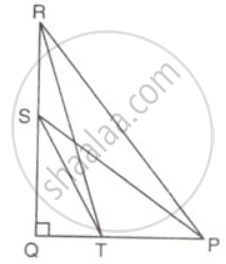# In the given figure, ∠Q = 90°, PS is a median om QR from P, and RT divides PQ in the ratio 1 : 2. Find: tan ∠ PSQ tan ∠ PRQ - Mathematics

Sum

In the given figure, ∠Q = 90°, PS is a median om QR from P, and RT divides PQ in the ratio 1 : 2. Find: ("tan" ∠"PSQ")/("tan"∠"PRQ")#### Solution

As PS is the median on QR from P.
∴ QS = SR
⇒ QR = 2QS
and RT divides PQ in the ratio 1 : 2
∴ QT = x and PT = 2x
⇒  PQ = 3x

("tan" ∠"PSQ")/("tan"∠"PRQ")

= ("PQ"/"QS")/("PQ"/"QR")

= "PQ"/"QS" xx "QR"/"PQ"

= (2"QS")/("QS")
= 2.

Concept: Reciprocal Relations
Is there an error in this question or solution?

#### APPEARS IN

Frank Class 9 Maths ICSE
Chapter 26 Trigonometrical Ratios
Exercise 26.1 | Q 19.1